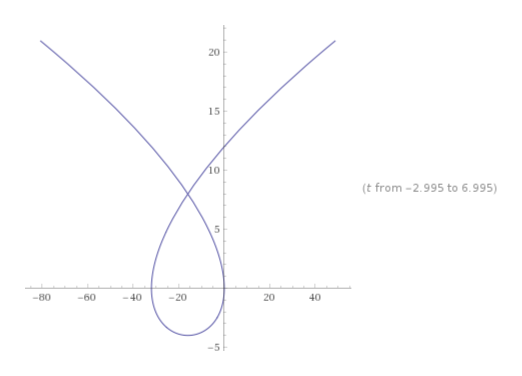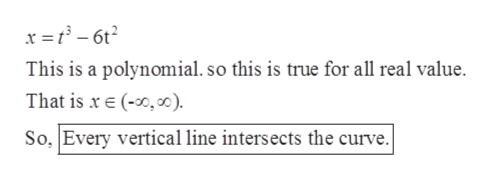# Give the parametric curve:x = t3-6t2y = t2-4tDoes every horizontal line intersect the curve? Does every vertical line intersect the curve? Explain shortly.

Question
4 views

Give the parametric curve:

x = t3-6t2

y = t2-4t

Does every horizontal line intersect the curve? Does every vertical line intersect the curve? Explain shortly.

check_circle

Step 1

Given: the parametric curve:

Step 2

The graph of the given parametric curve is given below:help_outlineImage Transcriptionclose20 15 10 (t from -2.995 to 6.995) -80 -60 -40 -20 20 40 -5- fullscreen
Step 3

Calculatio...help_outlineImage Transcriptionclosex = t – 6t? This is a polynomial. so this is true for all real value. That is x e (-0,). So, Every vertical line intersects the curve. fullscreen

### Want to see the full answer?

See Solution

#### Want to see this answer and more?

Solutions are written by subject experts who are available 24/7. Questions are typically answered within 1 hour.*

See Solution
*Response times may vary by subject and question.
Tagged in

### Calculus